## Parameters of Operational Amplifier:

Two Important Parameters of Operational Amplifier are the slew rate and the power supply rejection ratio (PSRR). Let us discuss and obtain the expressions for these two Parameters of Operational Amplifier

### Power Supply Rejection Ratio: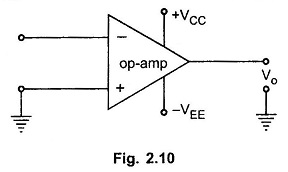The power supply rejection ratio (PSRR) is defined as the ratio of the change in input offset voltage due to the change in supply voltage producing it, keeping other power supply voltage constant. It is also called power supply sensitivity (PSV).

Now if VEE is constant and due to certain change in VCC , there is change in input offset voltage then PSRR is defined as,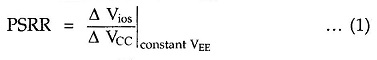For a fixed VCC, if there is a change in VEE then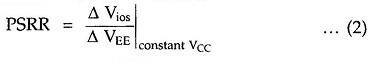As input offset voltage is very small, PSRR is expressed in mV/V or μV/V. The typical value of PSRR for IC 741 Op-amp is 30 µV/V.

### Slew Rate Definition and Formula:

The slew rate is defined as the maximum rate of change of output voltage with time.

The slew rate is specified in V/μ sec. Thus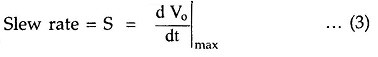The slew rate is caused due to limited charging rate of the compensating capacitor and current limiting and saturation of the internal stages of an op-amp, when a high frequency, large amplitude signal is applied. The internal capacitor voltage cannot change instantaneously.

For large charging rate, the capacitor should be small or charging current should be large.

Hence by the Parameters of Operational Amplifier slew rate for the op-amp whose maximum internal capacitor charging current is known, can be obtained as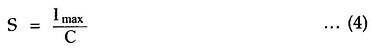For example, for IC 741 the charging current is 15 μA and the internal capacitor is 30 pF, hence its slew rate is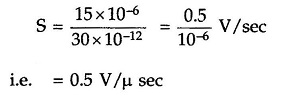The slew rate for noninverting amplifier is worst (smallest) and the circuit used for its measurement is as shown in the Fig. 2.11.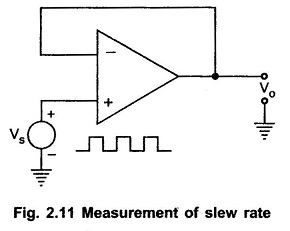The square wave input is applied to the circuit. The frequency of the applied input is increased till the distorted output is observed on the oscilloscope. It is shown in the Fig. 2.12 (a) and (b).

Then the slew rate is ΔVo/Δt.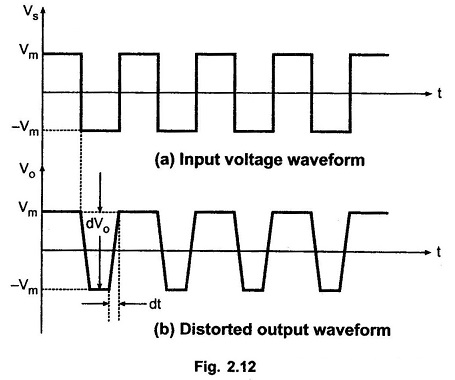For example, if the voltage rises from -6 to +6 in 24 μsec then the slew rate is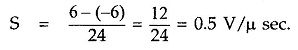The typical value of the slew rate for IC 741 Op-amp is 0.5 V/μsec, ideally it should be infinite. Higher the value of slew rate, better is the performance of op-amp.

Scroll to Top